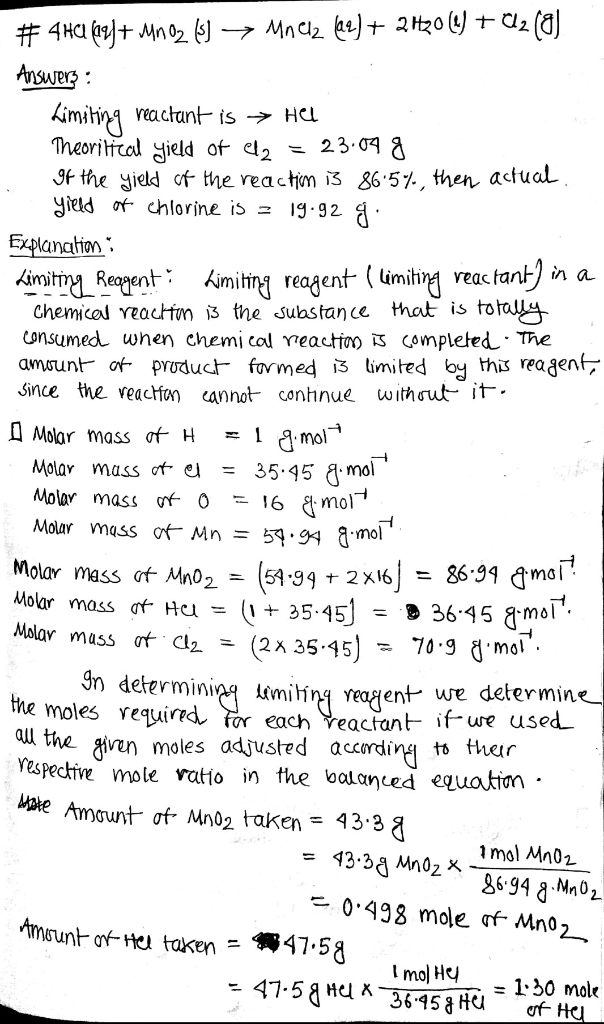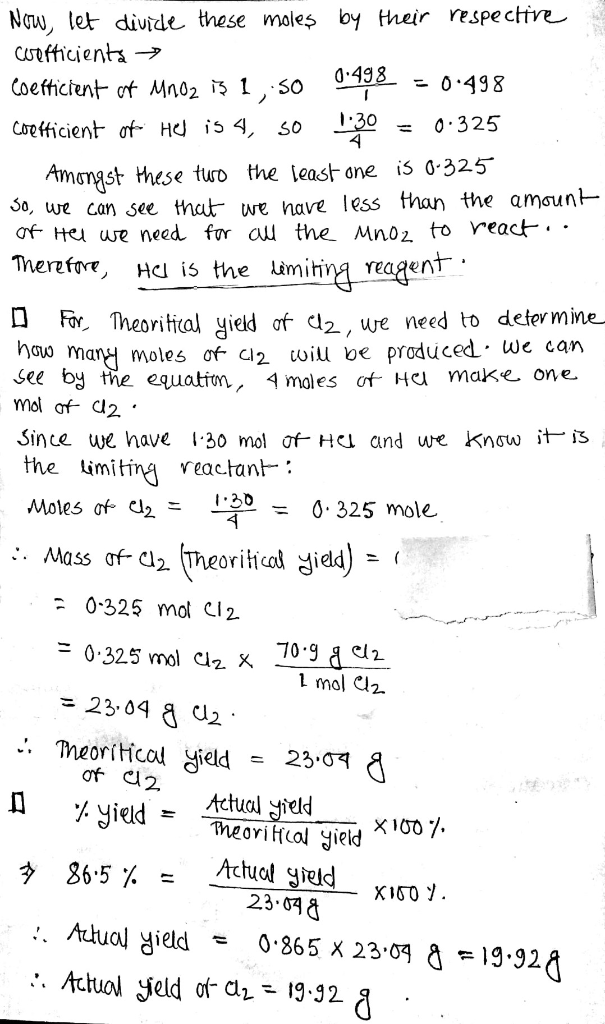In: Chemistry

# Chlorine gas can be prepared in the laboratory by the reaction of hydrochloric acid with manganese(IV)...

Chlorine gas can be prepared in the laboratory by the reaction of hydrochloric acid with manganese(IV) oxide.

4 HCl ( aq ) + MnO 2 ( s ) ⟶ MnCl 2 ( aq ) + 2 H 2 O ( l ) + Cl 2 ( g )

A sample of 43.3 g MnO 2 is added to a solution containing 47.5 g HCl

. What is the limiting reactant?

MnO 2

or

HCl

What is the theoretical yield of Cl 2 ?

theoretical yield:

If the yield of the reaction is 86.5 % , what is the actual yield of chlorine?

actual yield:

## Solutions

##### Expert Solution## Related Solutions

##### Chlorine gas can be prepared in the laboratory by the reaction of hydrochloric acid with manganese(IV)...
Chlorine gas can be prepared in the laboratory by the reaction of hydrochloric acid with manganese(IV) oxide. 4HCl(aq)+MnO2(s)⟶MnCl2(aq)+2H2O(l)+Cl2(g) A sample of 41.5 g MnO2 is added to a solution containing 48.7 g HCl. What is the theoretical yield of Cl2? theoretical yield:___gCl2 If the yield of the reaction is 76.3%, what is the actual yield of chlorine? actual yield:___g Cl2
##### Chlorine gas can be prepared in the laboratory by the reaction of hydrochloric acid with manganese(IV)...
Chlorine gas can be prepared in the laboratory by the reaction of hydrochloric acid with manganese(IV) oxide: 4HCL(aq)+MnO2(s)------>MnCl2(aq)+2H2O(l)+Cl2(g) You ad 39.3g of MnO2 to a solution containing 49.3g of HCl a. what is the limiting reactant? b. what is the theoretical yield of Cl2? c. if the yield of the reaction is 84.1%, what is the actual yield of chlorine?
##### Chlorine can be prepared in the laboratory by the reaction of manganese dioxide with hydrochloric acid,...
Chlorine can be prepared in the laboratory by the reaction of manganese dioxide with hydrochloric acid, HCl(aq), as described by the chemical equation MnO2(s)+4HCl(aq)⟶MnCl2(aq)+2H2O(l)+Cl2(g) How much MnO2(s) should be added to excess HCl(aq) to obtain 265 mL Cl2(g) at 25 °C and 725 Torr?
##### Chlorine can be prepared in the laboratory by the reaction of manganese dioxide with hydrochloric acid,...
Chlorine can be prepared in the laboratory by the reaction of manganese dioxide with hydrochloric acid, HCl(aq), as described by the chemical equation: MnO2(s) + 4HCl --> MnCl2 (aq) + 2H2O (l) + Cl2 (g) How much MnO2(s) should be added to excess HCl(aq) to obtain 405 mL of Cl2(g) at 25 �C and 805 Torr?
##### chlorine gas can be prepared in the lab by the reaction of hydrochloric acid with manganese...
chlorine gas can be prepared in the lab by the reaction of hydrochloric acid with manganese oxide. you add 42.5g of MnO2 to a solution containing 48.9g of HCL. A-what is the theoretical yield of Cl2? B- if the yield of the reaction is 79.5%, what is the actual yield of chlorine?
##### Chlorine gas (Cl2) can be produced by reaction of hydrochloride acid with manganese oxide. What is...
Chlorine gas (Cl2) can be produced by reaction of hydrochloride acid with manganese oxide. What is the volume of chlorine gas is produced by adding 1 g of MnO2 to one litre of 1 M HCl? MnO2 + 4HCl -> MnCl2 + Cl2 + 2H2O How much chlorine gas is produced when instead of 1 g MnO2 this time 50 g MnO2 is added?
##### 2. In manufacture of chlorine, feed containing hydrochloric acid gas and air are fed to the...
2. In manufacture of chlorine, feed containing hydrochloric acid gas and air are fed to the reactor. The product gases leaving the reactor are found to contain 13.2% HCl, 6.3% 02, 42.9% N2, 30% Cl2 and 7.6% H2O (by weight). Calculate: i) The percent excess air used. ii) The composition of gases entering reactor iii) The degree of completion of oxidation (conversion) (Hint: Start solving by change the mass flow rate to mol flow rate first.)
##### Write a balanced net ionic equation for the reaction of manganese(II) hydroxide, Mn(OH)2, with hydrochloric acid....
Write a balanced net ionic equation for the reaction of manganese(II) hydroxide, Mn(OH)2, with hydrochloric acid. Mn(OH)2(s) + 2H+(aq) ---> Mn2+(aq) + 2H2O(l) Mn(OH)2(s) + 2H+(aq) ---> MnH2(s) + 2OH−(aq) (OH)3(aq) + 3H+(aq) ---> 3H2O(l) 3OH−(aq) + 3H+(aq) ---> 3H2O(l) Mn2+(aq) + 2Cl−(aq) ---> MnCl2(aq)
##### For the following reaction, 3.46 grams of hydrochloric acid are mixed with excess oxygen gas. The...
For the following reaction, 3.46 grams of hydrochloric acid are mixed with excess oxygen gas. The reaction yields 0.728 grams of water. hydrochloric acid (aq) + oxygen (g) water (l) + chlorine (g) What is the theoretical yield of water ? What is the percent yield of water ?
##### Aqueous hydrochloric acid and aqueous potassium permanganate react to form chlorine gas, liquid water, solid potassium...
Aqueous hydrochloric acid and aqueous potassium permanganate react to form chlorine gas, liquid water, solid potassium chloride, and aqueous manganese chloride. If 8.88×104 milligrams of hydrochloric acid reacts with an excess of potassium permanganate, determine the mass of chlorine in grams. I really have no Idea how to approach this problem. Please help this is due at midnight tonight. A. 1.01×106 grams B. 7.79×103 grams C. 2.59×108 grams D. 5.53×105 grams E.54.0 grams